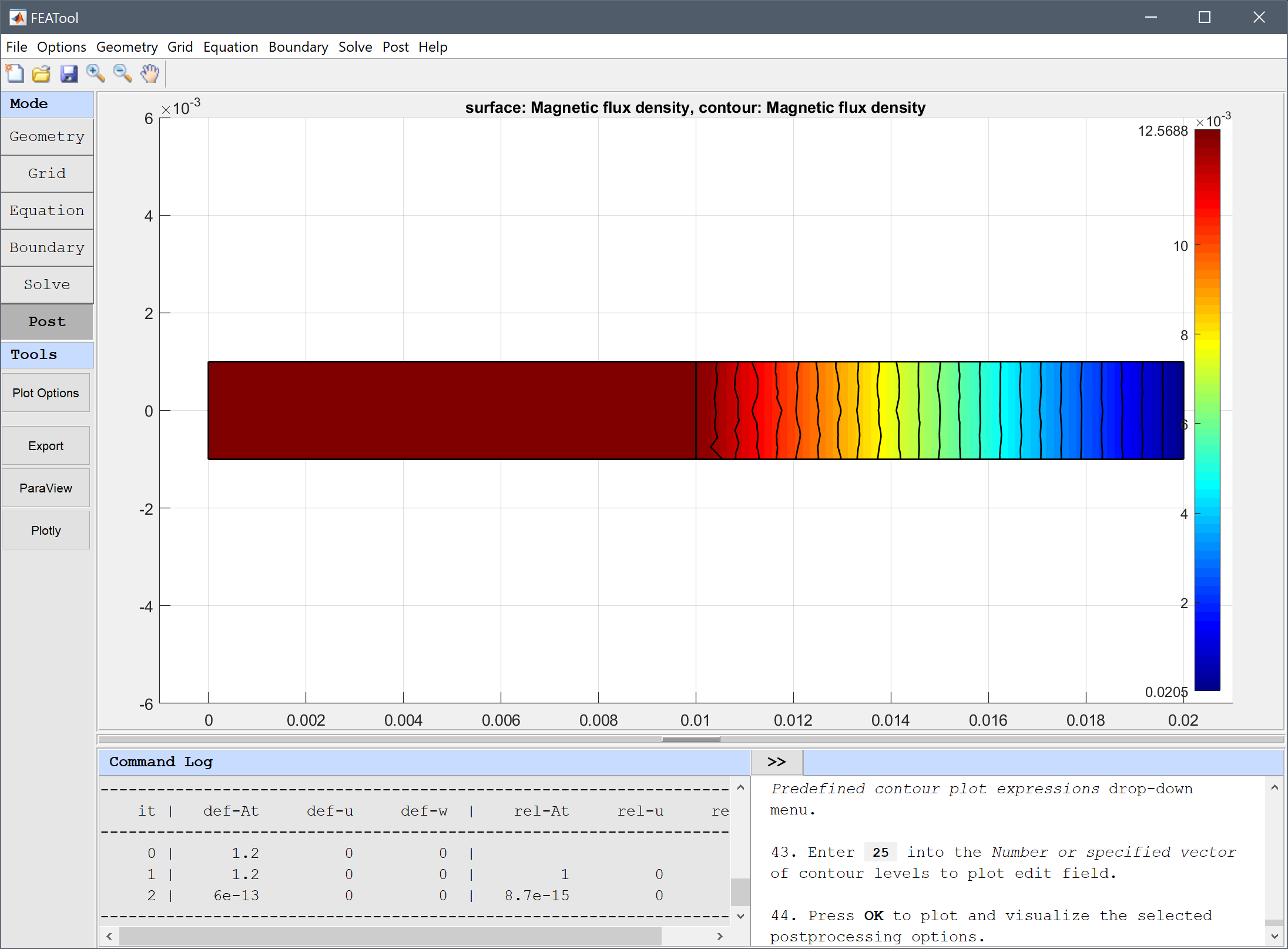# Stress Distribution in a Solenoid## Model Data

Physics Modes: axisymmetric stress strain magnetostatics

Keywords: solenoid magnetic force stress

This multiphysics model examines how magnetic forces give rise to stresses in a long thick cylindrical solenoid. A current density of 106 A/m2 is running through a wound coil with 1 cm inner to 2 cm outer radii. The material of the coil is assumed to have a modulus of elasticity of 1.075⋅1011 N/m2 and Poisson’s ratio of 0.33, and air occupies the inner hollow core.

As this is a one-way coupled problem, the magnetostatic effects will be solved for first, after which the calculated magnetic forces will be used as input to the stress-strain problem. Due to symmetry it is also sufficient to model a 2D axisymmetric cross-section. The resulting magnetic flux density and circumferential stress at r = 1.3 cm will be compared to the theoretical values of 8.798⋅10-3 T and 96.71 N/m, respectively .

This model is available as an automated tutorial by selecting Model Examples and Tutorials… > Multiphysics > Stress Distribution in a Solenoid from the File menu. Or alternatively, follow the linked step-by-step instructions.## Reference

 Moon FA. Magneto-Solid Mechanics, Chapter 4, John Wiley & Sons, NY, 1984.